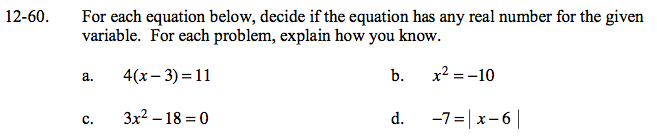### Home > GC > Chapter 12 > Lesson 12.2.1 > Problem12-60

12-60.
1. For each equation below, decide if the equation has any real number for the given variable. For each problem, explain how you know. Homework Help ✎

1. 4(x − 3) = 11

2. x2 = −10

3. 3x2 − 18 = 0

4. −7 = | x − 6 |Solve for x.
Is your solution a real number?

$4(x - 3) = 11$

$\underline{\:\:\:\:\:\:\:\:\div4\:\:\:\:\:\div4}$

$x - 3 = \frac{11}{4}$

$x = \frac{23}{4}$

Is it possible for the square or absolute value of a number to be negative?

No real solution, x² must be positive or zero.

Refer to part (a) for help.

Refer to part (b) for help.